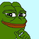1388 views
Linear Weighted MA that changes colors based on slope.

Green = slope up from last bar
Yellow = slope is 0 from last bar
Red = slope down from last bar

This time with the ability to change the period.
```//@version=2
study("LWMA w/ Color Change V1.1", shorttitle="LWMA", overlay=true)

p = input(defval=35, title="Period", minval=1)

ma = wma(close, p)

c = ma > ma ? green : ma < ma ? red : yellow

plot(ma, color=c)```